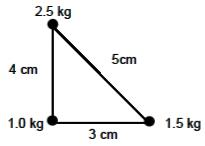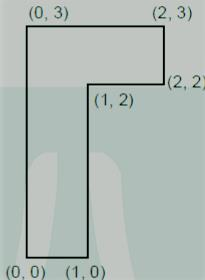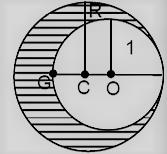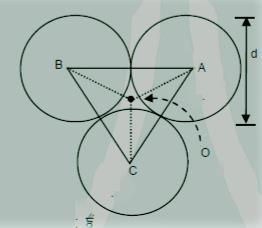Courses

# QUIZ 2:centre Of Mass,Linear Momentum,collisons(#Free Test Series)

## 15 Questions MCQ Test Physics chapterwise notes and mocks JEE | QUIZ 2:centre Of Mass,Linear Momentum,collisons(#Free Test Series)

Description
This mock test of QUIZ 2:centre Of Mass,Linear Momentum,collisons(#Free Test Series) for JEE helps you for every JEE entrance exam. This contains 15 Multiple Choice Questions for JEE QUIZ 2:centre Of Mass,Linear Momentum,collisons(#Free Test Series) (mcq) to study with solutions a complete question bank. The solved questions answers in this QUIZ 2:centre Of Mass,Linear Momentum,collisons(#Free Test Series) quiz give you a good mix of easy questions and tough questions. JEE students definitely take this QUIZ 2:centre Of Mass,Linear Momentum,collisons(#Free Test Series) exercise for a better result in the exam. You can find other QUIZ 2:centre Of Mass,Linear Momentum,collisons(#Free Test Series) extra questions, long questions & short questions for JEE on EduRev as well by searching above.
QUESTION: 1

Solution:
QUESTION: 2

Solution:
QUESTION: 3

### A machinist starts with three identical square plates but cuts one comer from one of them, two comers from the second and three comers from the third. Rank the three according to the x-coordinate of their centre of mass, from smallest to largest.Solution:
QUESTION: 4

A mass m is rest on an inclined plane of mass M which is further resting on a smooth horizontal plane. Now if the mass starts moving the position of C.M. of mass of system willSolution:
QUESTION: 5

A ball kept in a closed box moves in the box making collisions with the walls. The box is kept on a smooth surface. The velocity of the centre of mass

Solution:

As no external force acts on the (ball + box) system, hence velocity of the system remains constant.

*Answer can only contain numeric values
QUESTION: 6

A body of mass 5 kg explodes at rest into three fragments with masses in the ratio 1:1:3. The fragments with equal masses fly in mutually perpendicular directions with speeds of 21 m/s. What is the velocity (in m/s) of the heaviest fragment?(answer in rounded off upto two digits after decimal)

Solution:
*Answer can only contain numeric values
QUESTION: 7

Figure shows a cubical box that has been constructed from uniform metal plate of negligible thickness. The box is open at the top and has edge length 40 cm. The z co-ordinate of the centre of mass of the box in cm, is ___.Solution:
*Answer can only contain numeric values
QUESTION: 8

A highly elastic ball moving at a speed of 3 m/s approaches a wall moving towards it with a speed of 3 m/s. After the collision, what is the speed (in m/s) of the ball?Solution:
*Answer can only contain numeric values
QUESTION: 9

A ball impinges directly on another ball at rest. The first ball is brought to rest by the impact. If half of the kinetic energy is lost by the impact, the value of coefficient of restitution is _______.

Solution:
*Answer can only contain numeric values
QUESTION: 10

Two particles of equal masses moving with same speed collide perfectly inelastically. After the collision the combined mass moves with half of the speed of the individual masses. What is the angle (in degree) between the initial momenta of individual particle?

Solution:
QUESTION: 11

Three point particles of masses 1.0 kg, 1.5 kg and 2.5 kg are placed at three corners of a right angletriangle of sides 4.0 cm, 3.0 cm and 5.0 cm as shown in the figure. The center of mass of the system isat a point :Solution:
QUESTION: 12

Consider a uniform rod of mass M = 4m and length l pivoted about its centre. A mass m moving with
velocity v making angle θ=π/4 to the rod's long axis collides with one end of the rod and sticks to it. The
angular speed of the rod-mass system just after the collision is

Solution:
QUESTION: 13

The coordinates of centre of mass of a uniform flag shaped lamina (thin flat plate) of mass 4 kg. (The
coordinates of the same are shown in figure) areSolution:
QUESTION: 14

As shown in figure. When a spherical cavity (centred at O) of radius 1 is cut out of a uniform sphere of
radius R (centred at C), the centre of mass of remaining (shaded) part of sphere is at G, i.e on the
surface of the cavity. R can be determined by the equationSolution:
QUESTION: 15

Three solid spheres each of mass m and diameter d are stuck together such that the lines connecting
the centres form an equilateral triangle of side of length d. The ratio I0/IA of moment of inertial IA of the
system about an axis passing the centroid and about center of any of the spheres Iand perpendicular
to the plane of the triangle isSolution: